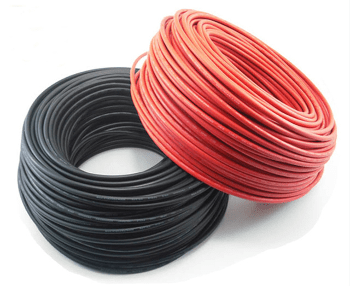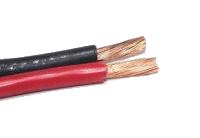# Why is it so important to size a cable for a solar system?

 If you have a solar system it is important to know how to size a cable. Why is that so? Because a solar system without cables can be compared to a really efficient machine which takes up space and does no one good. Cables are critical in solar systems as they are used in deploying electrical energy converted from the sun by the solar controller to the end devices and processes being powered by this energy.As important as cables are to solar systems, the sizes of these cables could mean the difference between an efficient solar system and one which is a security hazard waiting to happen due to overload.

### Calculating the cable size/thickness/diameter for a solar system

 Let us say for example that we are about to run a solar system which will power one led bulb streetlight.The streetlight is 11m (36 ft.) away from the solar controller, it will, therefore, imply that the longest cable running to and fro from the solar controller will be 22m (72.2 ft.).(Lx I x 0.04) ÷ (V ÷ 20) = CTWhere:
L = Cable length in meters (one meter is 3.3 feet)
I = Current in amps
V = System voltage (e.g. 12V, 24V or 48V)
CT = Cross-sectional area of the cable in mm² (what we are solving for)

For purposes of demonstration and to enable us to reach a reasonably applicable solution, we shall calculate the required cable thickness for the led streetlight scenario at 12V, 24V and 48V.
Calculating required cable thickness at 12V
(22m x 14.08A x 0.04) ÷ (12V ÷ 20) = 20.65mm²
Here is the same calculation for a 24-volt system:
(22m x 7.04A x 0.04) ÷ (24V ÷ 20) = 5.15mm²
And at 48V
(22m x 3.52A x 0.04) ÷ (48V ÷ 20) = 1.63mm²At 12V, the thickness required of our cables are so great that we cannot practically run such a system. The available standard cable nearest to that which we need to run our 12V solar powered streetlight of 20.65mm2 is the AWG 4 cable with a diameter of 5.19mm.Cable this size is heavy, thick, inflexible, very expensive and hard to source. This implies the need to lay very thick cables for our streetlights, which is so impractical we might as well return to the coal-age.
Realistically, due to cable sizing, we are going to need to use either 24V or 48V for all the reasons why we can’t use the 12V system.